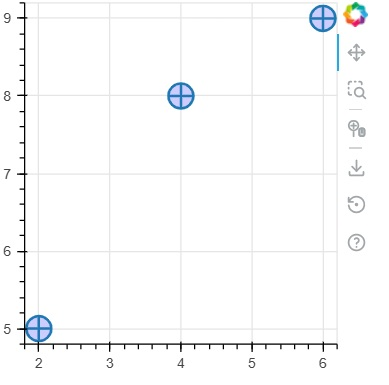# Bokeh - Circle Glyphs

The figure object has many methods using which vectorised glyphs of different shapes such as circle, rectangle, polygon, etc. can, be drawn.

Following methods are available for drawing circle glyphs

## circle()

The circle() method adds a circle glyph to the figure and needs x and y coordinates of its center. Additionally, it can be configured with the help of parameters such as fill_color, line-color, line_width etc.

## circle_cross()

The circle_cross() method adds circle glyph with a ‘+’ cross through the center.

## circle_x()

The circle_x() method adds circle with an ‘X’ cross through the center.

## Example

Following example shows use of various circle glyphs added to Bokeh figure −

```from bokeh.plotting import figure, output_file, show
plot = figure(plot_width = 300, plot_height = 300)
plot.circle(x = [1, 2, 3], y = [3,7,5], size = 20, fill_color = 'red')
plot.circle_cross(x = [2,4,6], y = [5,8,9], size = 20, fill_color = 'blue',fill_alpha = 0.2, line_width = 2)
plot.circle_x(x = [5,7,2], y = [2,4,9], size = 20, fill_color = 'green',fill_alpha = 0.6, line_width = 2)
show(plot)
```

## Output